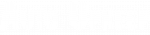# MPG Calculation

Q: How do I calculate the fuel efficiency of my car?

A: To calculate how many miles per gallon (MPG) your car is achieving divide the number of miles traveled by the number of gallons used. For example, if you traveled 300 miles and used 15 gallons of gasoline, your car achieved 20 MPG.